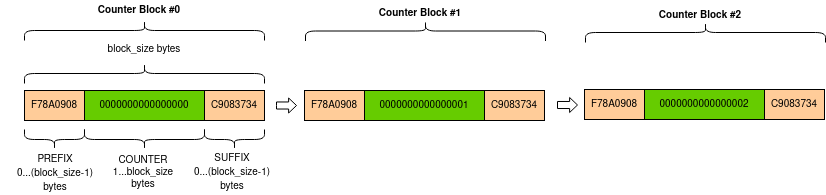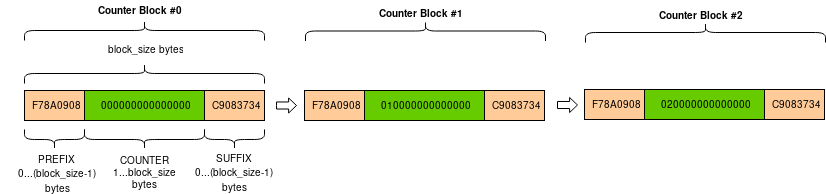# Crypto.Util package¶

Useful modules that don’t belong in any other package.

## Crypto.Util.Padding module¶

This module provides minimal support for adding and removing standard padding from data. Example:

>>> from Crypto.Util.Padding import pad, unpad
>>> from Crypto.Cipher import AES
>>> from Crypto.Random import get_random_bytes
>>>
>>> data = b'Unaligned'   # 9 bytes
>>> key = get_random_bytes(32)
>>> iv = get_random_bytes(16)
>>>
>>> cipher1 = AES.new(key, AES.MODE_CBC, iv)
>>> ct = cipher1.encrypt(pad(data, 16))
>>>
>>> cipher2 = AES.new(key, AES.MODE_CBC, iv)
>>> pt = unpad(cipher2.decrypt(ct), 16)
>>> assert(data == pt)

Crypto.Util.Padding.pad(data_to_pad, block_size, style='pkcs7')

Apply standard padding.

Parameters: data_to_pad (byte string) – The data that needs to be padded. block_size (integer) – The block boundary to use for padding. The output length is guaranteed to be a multiple of block_size. style (string) – Padding algorithm. It can be ‘pkcs7’ (default), ‘iso7816’ or ‘x923’. the original data with the appropriate padding added at the end. byte string
Crypto.Util.Padding.unpad(padded_data, block_size, style='pkcs7')

Remove standard padding.

Parameters: padded_data (byte string) – A piece of data with padding that needs to be stripped. block_size (integer) – The block boundary to use for padding. The input length must be a multiple of block_size. style (string) – Padding algorithm. It can be ‘pkcs7’ (default), ‘iso7816’ or ‘x923’. data without padding. byte string ValueError – if the padding is incorrect.

## Crypto.Util.RFC1751 module¶

Crypto.Util.RFC1751.english_to_key(s)

Transform a string into a corresponding key.

Example:

>>> from Crypto.Util.RFC1751 import english_to_key
>>> english_to_key('RAM LOIS GOAD CREW CARE HIT')
b'66666666'

Parameters: s (string) – the string with the words separated by whitespace; the number of words must be a multiple of 6. A byte string.
Crypto.Util.RFC1751.key_to_english(key)

Transform an arbitrary key into a string containing English words.

Example:

>>> from Crypto.Util.RFC1751 import key_to_english
>>> key_to_english(b'66666666')
'RAM LOIS GOAD CREW CARE HIT'

Parameters: key (byte string) – The key to convert. Its length must be a multiple of 8. A string of English words.

## Crypto.Util.strxor module¶

Fast XOR for byte strings.

Crypto.Util.strxor.strxor(term1, term2, output=None)

XOR two byte strings.

Parameters: term1 (bytes/bytearray/memoryview) – The first term of the XOR operation. term2 (bytes/bytearray/memoryview) – The second term of the XOR operation. output (bytearray/memoryview) – The location where the result must be written to. If None, the result is returned. If output is None, a new bytes string with the result. Otherwise None.
Crypto.Util.strxor.strxor_c(term, c, output=None)

XOR a byte string with a repeated sequence of characters.

Parameters: term (bytes/bytearray/memoryview) – The first term of the XOR operation. c (bytes) – The byte that makes up the second term of the XOR operation. output (None or bytearray/memoryview) – If not None, the location where the result is stored into. If output is None, a new bytes string with the result. Otherwise None.

## Crypto.Util.Counter module¶

Richer counter functions for CTR cipher mode.

CTR is a mode of operation for block ciphers.

The plaintext is broken up in blocks and each block is XOR-ed with a keystream to obtain the ciphertext. The keystream is produced by the encryption of a sequence of counter blocks, which all need to be different to avoid repetitions in the keystream. Counter blocks don’t need to be secret.

The most straightforward approach is to include a counter field, and increment it by one within each subsequent counter block.

The new() function at the module level under Crypto.Cipher instantiates a new CTR cipher object for the relevant base algorithm. Its parameters allow you define a counter block with a fixed structure:

• an optional, fixed prefix
• the counter field encoded in big endian mode

The length of the two components can vary, but together they must be as large as the block size (e.g. 16 bytes for AES).

Alternatively, the counter parameter can be used to pass a counter block object (created in advance with the function Crypto.Util.Counter.new()) for a more complex composition:

• an optional, fixed prefix
• the counter field, encoded in big endian or little endian mode
• an optional, fixed suffix

As before, the total length must match the block size.

The counter blocks with a big endian counter will look like this:The counter blocks with a little endian counter will look like this:Example of AES-CTR encryption with custom counter:

from Crypto.Cipher import AES
from Crypto.Util import Counter
from Crypto import Random

nonce = Random.get_random_bytes(4)
ctr = Counter.new(64, prefix=nonce, suffix=b'ABCD', little_endian=True, initial_value=10)
key = b'AES-128 symm key'
plaintext = b'X'*1000000
cipher = AES.new(key, AES.MODE_CTR, counter=ctr)
ciphertext = cipher.encrypt(plaintext)

Crypto.Util.Counter.new(nbits, prefix='', suffix='', initial_value=1, little_endian=False, allow_wraparound=False)

Create a stateful counter block function suitable for CTR encryption modes.

Each call to the function returns the next counter block. Each counter block is made up by three parts:

 prefix counter value postfix

The counter value is incremented by 1 at each call.

Parameters: nbits (integer) – Length of the desired counter value, in bits. It must be a multiple of 8. prefix (byte string) – The constant prefix of the counter block. By default, no prefix is used. suffix (byte string) – The constant postfix of the counter block. By default, no suffix is used. initial_value (integer) – The initial value of the counter. Default value is 1. little_endian (boolean) – If True, the counter number will be encoded in little endian format. If False (default), in big endian format. allow_wraparound (boolean) – This parameter is ignored. An object that can be passed with the counter parameter to a CTR mode cipher.

It must hold that len(prefix) + nbits//8 + len(suffix) matches the block size of the underlying block cipher.

## Crypto.Util.number module¶

Crypto.Util.number.GCD(x, y)

Greatest Common Denominator of x and y.

Crypto.Util.number.bytes_to_long(s)

Convert a byte string to a long integer (big endian).

In Python 3.2+, use the native method instead:

>>> int.from_bytes(s, 'big')


For instance:

>>> int.from_bytes(b'P', 'big')
80


This is (essentially) the inverse of long_to_bytes().

Crypto.Util.number.ceil_div(n, d)

Return ceil(n/d), that is, the smallest integer r such that r*d >= n

Crypto.Util.number.getPrime(N, randfunc=None)

Return a random N-bit prime number.

If randfunc is omitted, then Random.get_random_bytes() is used.

Crypto.Util.number.getRandomInteger(N, randfunc=None)

Return a random number at most N bits long.

If randfunc is omitted, then Random.get_random_bytes() is used.

Deprecated since version 3.0: This function is for internal use only and may be renamed or removed in the future. Use Crypto.Random.random.getrandbits() instead.

Crypto.Util.number.getRandomNBitInteger(N, randfunc=None)

Return a random number with exactly N-bits, i.e. a random number between 2**(N-1) and (2**N)-1.

If randfunc is omitted, then Random.get_random_bytes() is used.

Deprecated since version 3.0: This function is for internal use only and may be renamed or removed in the future.

Crypto.Util.number.getRandomRange(a, b, randfunc=None)

Return a random number n so that a <= n < b.

If randfunc is omitted, then Random.get_random_bytes() is used.

Deprecated since version 3.0: This function is for internal use only and may be renamed or removed in the future. Use Crypto.Random.random.randrange() instead.

Crypto.Util.number.getStrongPrime(N, e=0, false_positive_prob=1e-06, randfunc=None)

Return a random strong N-bit prime number. In this context, p is a strong prime if p-1 and p+1 have at least one large prime factor.

Parameters: N (integer) – the exact length of the strong prime. It must be a multiple of 128 and > 512. e (integer) – if provided, the returned prime (minus 1) will be coprime to e and thus suitable for RSA where e is the public exponent. false_positive_prob (float) – The statistical probability for the result not to be actually a prime. It defaults to 10-6. Note that the real probability of a false-positive is far less. This is just the mathematically provable limit. randfunc (callable) – A function that takes a parameter N and that returns a random byte string of such length. If omitted, Crypto.Random.get_random_bytes() is used. The new strong prime.

Deprecated since version 3.0: This function is for internal use only and may be renamed or removed in the future.

Crypto.Util.number.inverse(u, v)

The inverse of u mod v.

Crypto.Util.number.isPrime(N, false_positive_prob=1e-06, randfunc=None)

Test if a number N is a prime.

Parameters: false_positive_prob (float) – The statistical probability for the result not to be actually a prime. It defaults to 10-6. Note that the real probability of a false-positive is far less. This is just the mathematically provable limit. randfunc (callable) – A function that takes a parameter N and that returns a random byte string of such length. If omitted, Crypto.Random.get_random_bytes() is used. True is the input is indeed prime.
Crypto.Util.number.long_to_bytes(n, blocksize=0)

Convert an integer to a byte string.

In Python 3.2+, use the native method instead:

>>> n.to_bytes(blocksize, 'big')


For instance:

>>> n = 80
>>> n.to_bytes(2, 'big')
b'P'


If the optional blocksize is provided and greater than zero, the byte string is padded with binary zeros (on the front) so that the total length of the output is a multiple of blocksize.

If blocksize is zero or not provided, the byte string will be of minimal length.

Crypto.Util.number.size(N)

Returns the size of the number N in bits.